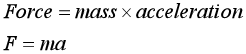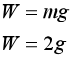# Explain Newton's second Law and solve related problemsGiven a mass of 2.00 kg. Let g = the acceleration due to gravity on Earth in SI units. Which of these expressions will yield the weight of the mass on Earth in SI units? a.zero b.4g c.2g d.0.25g e.0.20g

Question
64 views

Explain Newton's second Law and solve related problems

Given a mass of 2.00 kg. Let g = the acceleration due to gravity on Earth in SI units. Which of these expressions will yield the weight of the mass on Earth in SI units?

 a. zero b. 4g c. 2g d. 0.25g e. 0.20g
check_circle

Step 1

Newton’s second law of motion

Newton’s second law of motion states that the acceleration of the object is directly proportional to the net force acting on it and will be inversely proportional to the mass of the object.The direction of the acceleration will be in the direction of the net forceStep 2

Given

Mass m = 2 kg

Step 3

Solution

The weight of an object is the gravitational force exerted by the earth on the object. It is proportional to the mass of the object...

### Want to see the full answer?

See Solution

#### Want to see this answer and more?

Solutions are written by subject experts who are available 24/7. Questions are typically answered within 1 hour.*

See Solution
*Response times may vary by subject and question.
Tagged in

### Physics